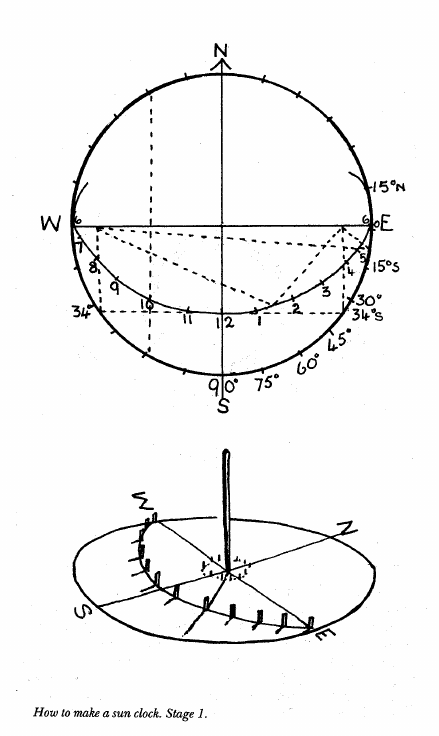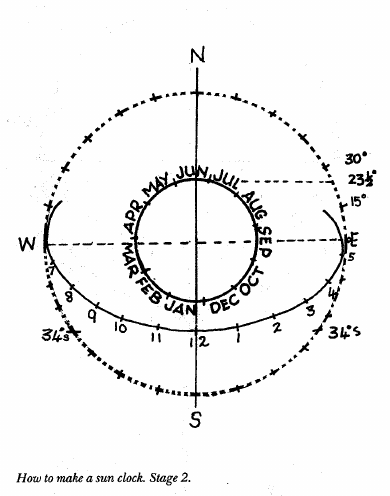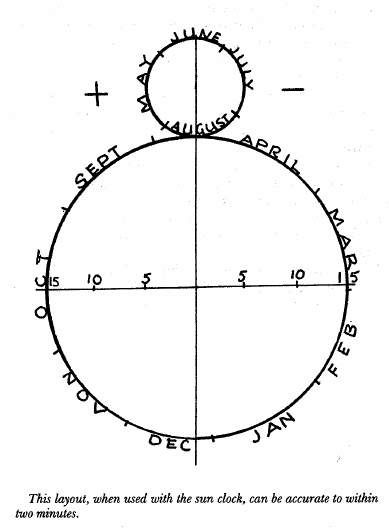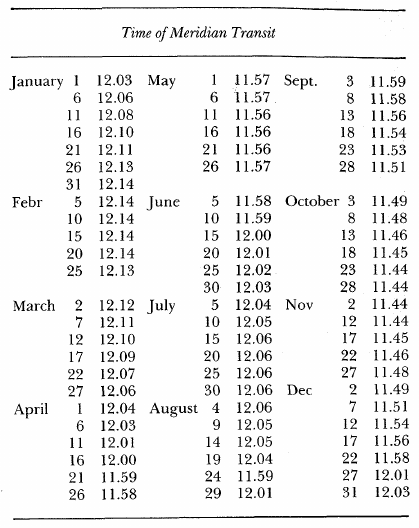## Camp Sun Clock

Select a patch of bare earth near the camp. It must be level and open to the sun all day. Stick a peg in the centre of this patch and, with a length of cord as a loop around the peg, scratch a circle on the ground.

This must be at least 1.5 metres across. From the peg, which is now the centre of the circle, carefully draw a line true north. This must be accurately true north and not magnetic north. Extend this line to cut the southern side of the circle, and then draw in accurate east-west lines crossing at the circle's centre.

Divide the circumference of the circle into 24 equal divisions. Each of these divisions will be 15".

Now have a look at your map and find out what degree of latitude you are in. Measure this degree on the outside circle working from where it is cutting the east-west line. Put a peg on each side of the circle's edge to mark the latitude degrees.

Be careful to note whether your latitude is north or south of the equator. Stretch the cord over the two pegs and mark where it crosses the north-south line. Now put a peg on the north-south line where the cord crosses it.

Next put two other pegs at either end of the east-west line so that the 'degree' pegs on the circle are at right angles. Tie a cord to each of these pegs and have the cord pass round the peg on the north-south line. Lift the cord over the centre peg and with the point of a knife scratch an ellipse on the ground so that it touches the circle where the east-west line crosses and also touches the point on the north-south line where the peg is.

Connect up the 15 degree marks on the circle by means of the cord.

Where the cord crosses the ellipse put a very small peg very firmly into the ground.

There will be 13 of these pegs and they will follow the curve of the ellipse. These are the hour pegs, starting from 6 a.m. on the left, where the west line cuts the circle, 12 noon on the north-south line and 6 p.m. on the right where the east line cuts the circle.true northYou then determine where to place the shadow stick. This depends on the sun's position north or south of the equator.

Draw another circle inside the big circle using the same centre. The radius of this circle must be equal to 23% degrees of the big one. Cut this inner circle into 12 equal divisions and mark June at the north side and follow on with July, August and so on clockwise. Then divide June into four equal divisions and do the same with December at the south end. Offset all divisions one fourth in a clockwise direction.

The north-south line will now pass through the third division of June and December. Put pegs in for each of the 12 month's divisions.

T o find the sun's position at any time of the year draw a line from the month, and approximate day thereof, to thenorth-south line. This must parallel the east-west line.

Where this line cuts the north-south line is where you place your shadow stick.

To get absolutely reliable time from the sun, two corrections for longitude and for the 'equation of time' are required (see page 302).

Equation of time - and corrections to standard time (page 302)

Each day every longtitude of the earth passes under the sun, but because of the slight variation in the earth's path, the exact moment when the sun passes over the meridian of longitude is not precisely at twelve o'clock every day. The difference may be as much as 16 minutes of time before twelve o'clock on your clock time and fourteen minutes after twelve o'clock.

This passage of the sun over the imaginary north-south line is called 'meridian transit' and as you will see it differs from clock time throughout the course of the year, except for four days (16 April, 15June, 30 August, 25 December).

For convenience, the time of meridian transit is averaged out over the year, and the average is called 'mean' time. The sun's passage of the meridian time is called 'solar' (sun) time. The correction of time of the two is called 'equation of time.'

The following simple table on meridian transit can be shown in the form of the figure '8' for your easy memorising.A figure eight drawn to the proportions shown and with the four dates remembered when meridian transit coincides with mean time will give reasonably accurate corrections.

Note: The four dates when there is no correction are 16 April, 15June, 30 August and 25 December.

The top section of the figure 8 is about one-third the size of the lower half. T h e horizontal line is divided into three five-minute sections to right and left, and the right side marked plus to mean that the sun is ahead of mean time. The left is marked minus, the sun is behind mean time.

The application of this 'equation of time' correction will be required if you want to get accurate time from the sun, and also for correction to the compass-sun clock.

The 'shadow' reading with these corrections will be right to two minutes if your north-south line has been accurate.

If west of the meridian of standard time add four minutes to sun clock time for each degree. If east of the meridian deduct four minutes for each degree.

Draw a figure 8 near the sun clock on the ground with the top half of the '8' just less than one third of the size of the bottom half. Draw a line across the middle of the bottom half of the '8' and cut it into three equal divisions on each side of a centre line.

Each of these divisions represents five minutes of time.

Now mark off the figure '8' into approximate divisions (see illustration). Put pegs in the ground to mark these divisions and also the five-minute divisions on the cross line.

Put a minus sign on the right hand corner and a plus sign on the left.

Minus means that the sun time is behind clock time.

Therefore you must add. Plus means that the sun time is ahead of clock time.

SOURCE: Australian Bushcraft - Richard Graves  1984